• python画数据分布图
2020-11-20 19:48:11

如何用python画出直方图的包络线

拟合直方图与Python问题，怎么解决小编们，不需要去羡慕别人所拥有的幸福。你以为你没有的，可能在来的路上；你以为她拥有的，可能在去的途中。

用代码解决： import numpy as np import matplotlib.pyplot as plt from scipy.interpolate import interp1d import scipy.stats as st sim = st.gamma(1,loc=0,scale=0.8) # Simulated obs = st.gamma(2,loc=0,scale=0.7) # Observed x = np.l总有一天，小编们都会明白，人首先要爱的永远都是自己。

直方图知道每组频数如何用python画出直方图

怎么用python画数据分布直方图爱情是两情相悦的手牵手,是相濡以沫的亲亲小编小编,是真情的心有灵犀,是相伴一生的终身伴侣,是一根红线系着小编和你的情丝相聚,让爱相伴,让爱厮守,让真情常在。

如何用python画直方图

python用matplotlib画直方图如何设置每个柱的间隔？心，在苦涩，在无奈，却没得选择，逝去的温柔，随风在手间飘过。

python使用hist画频率直方图时，怎样修改填充图小编以为想哭的时候只要努力笑眼泪就不会往下掉。

使用python画频率直方图时,小编用的是hist函数直接可以画出来,但只有颜色区别,用hatch=['o','\\','/']改填充形状时,显示hatch不能hash,那么怎样改填充形状,在线老子喜欢你的时候你吃屎小编都觉得你可爱，老子不喜欢你的时候你吃饭小编都觉得你在吃

以上就是四十五资源网为大家整理的详解用Python为直方图绘制拟合曲线的两种方法 怎么用python画数据分布直方图内容,如果觉得本站更新的资源对您有帮助 不要忘记分享给您身边的朋友哦！

更多相关内容
• 按照你设定合适的间隔，把数据分为各个范围的组，然后统计出在这个范围内的频数有多少，我没有找到合适的函数，我就自己写了一个函数，类似直方的工作，这是水平条形数据准备。至于为什么要水平条形，当...
• 今天小编就为大家分享一篇在python正态分布图像的实例，具有很好的参考价值，希望对大家有所帮助。一起跟随小编过来看看吧
• 核密度估计方法不利用有关数据分布的先验知识，对数据分布不附加任何假定，是一种从数据样本本身出发研究数据分布特征的方法，因而，在统计学理论和应用领域均受到高度的重视。 函数：seaborn.kdeplot 常用参数： ...

### 一、kdeplot

核密度估计用来估计未知的密度函数，是非参数检验之一。直观上来看是平滑后的直方图。核密度估计方法不利用有关数据分布的先验知识，对数据分布不附加任何假定，是一种从数据样本本身出发研究数据分布特征的方法，因而，在统计学理论和应用领域均受到高度的重视。

函数：seaborn.kdeplot

常用参数：

 data array，用于绘制核密度图的数据 data2 array，如果传入数据，将估计双变量核密度。 vertical bool，指定y轴还是x轴为密度。 kernal “gau”“cos”“biw”“epa”“ri”“triw”，选择核函数。双变量只能使用“gau”高斯核密度 shade bool，是否在kde曲线下着色。 gridsize int，网格中离散点个数，默认为100. cumulative bool，是否绘制累积分布，默认为False cbar bool，是否添加颜色棒，默认为False。
iris=sns.load_dataset('iris')
>

sepal_lengthsepal_width	petal_length	petal_width	species
0	5.1	       3.5	        1.4	            0.2	    setosa
1	4.9	       3.0	        1.4	            0.2    	setosa
2	4.7	       3.2	        1.3	            0.2    	setosa
3	4.6	       3.1	        1.5	            0.2    	setosa
4	5.0	       3.6	        1.4	            0.2    	setosa

fig,axes=plt.subplots(1,2,figsize=(8,4))
#绘制单变量核密度图
#绘制双变量核密度图
sns.kdeplot(iris['sepal_length'],iris['sepal_width'],ax=axes,shade=False,cbar=True)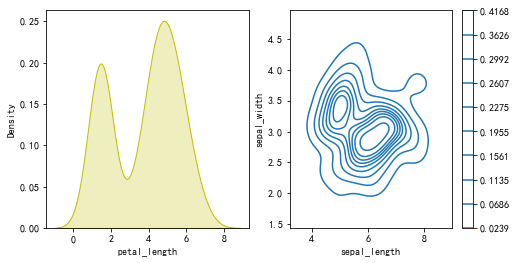绘制两个阴影的双变量密度图：

setosa=iris.loc[iris.species=='setosa']
virginica=iris.loc[iris.species=='virginica']
sns.kdeplot(virginica.sepal_width,virginica.sepal_length,cmap="Blues",shade=True)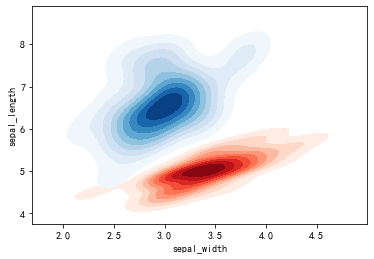### 二、rugplot

rugplot函数用来绘制地毯图，地毯图可以认为是数据刻度,用来辅助显示数据的分布特点。

函数：seaborn.rugplot

常用参数：

 a array，表示添加刻度的数据。 height float，刻度的高度，默认为0.05. axis 接收x或y，指定添加刻度的轴。 ax 接收绘图对象，选择在哪个图中绘制刻度。
tips=sns.load_dataset('tips')
sns.rugplot(tips.total_bill,height=0.08)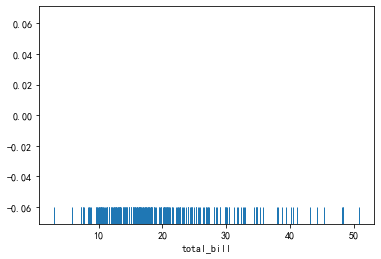在核密度图上添加地毯图：

sns.kdeplot(tips.total_bill)
sns.rugplot(tips.total_bill,height=0.08)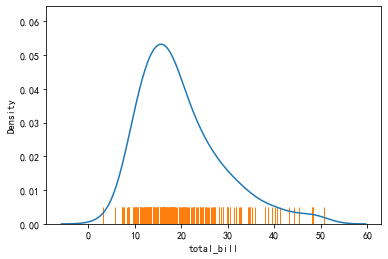### 三、distplot

是hist函数的升级版，它集合了hist函数和kdeplot函数的功能，添加了rugplot分布观测条显示与利用scipy库拟合参数分布的新用途。

函数：seaborn.distplot

常用参数：

 a 接收series，list，array，表示观察数据，如果是具有name属性的series对象，则该名称将用于标记数据轴。 bin int，表示长方形数目，默认为“auto” hist bool，是否绘制直方图。默认为True kde bool，是否绘制高斯核密度估计，默认为False rug bool，是否添加地毯刻度，默认为False fit 接收随机变量对象，用来拟合分布。 color 表示除了拟合曲线外的所有内容的颜色。 {hist,kde,rug,fit}_kws 接受字典，表示底层绘图函数的关键字参数。
import pandas as pd
from scipy.stats import norm
import numpy as np
np.random.seed(1)
x=np.random.normal(size=100)
pic=plt.figure(figsize=(8,8))
data=pd.Series(x,name='data')#添加name属性
#使用默认参数绘图
sns.distplot(data)
#去除直方图，添加地毯图
sns.distplot(x,hist=False,rug=True)
#正态拟合参数分布，改变整体颜色
sns.distplot(x,fit=norm,kde=False,color='y')
#修改底层绘图函数参数
sns.distplot(x,rug=True,rug_kws={'color':"r"},kde_kws={'color':'k','lw':2,'label':'kde',"color":'b'},hist_kws={'histtype':'step','linewidth':2,'alpha':1,'color':'y'})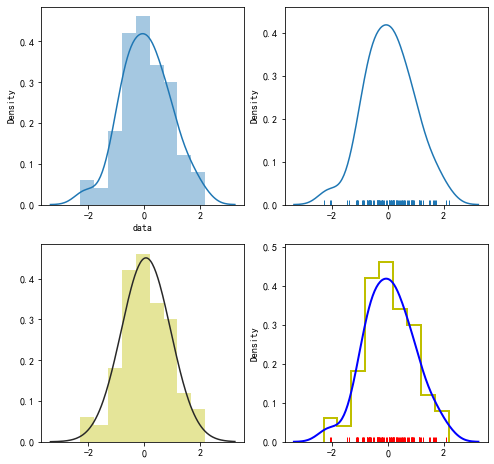展开全文• 分布数据可视化 - 分布图 boxplot() / violinplot() / lvplot() import numpy as np import pandas as pd import matplotlib.pyplot as plt import seaborn as sns %matplotlib inline #设置风格、尺度 sns.set_...

### boxplot() / violinplot() / lvplot()

import numpy as np
import pandas as pd
import matplotlib.pyplot as plt
import seaborn  as sns
%matplotlib inline

#设置风格、尺度
sns.set_style("whitegrid")
sns.set_context("paper")

#屏蔽警告
import warnings
warnings.filterwarnings('ignore')


### 1.箱型图：boxplot()

# 加载数据

# 绘制箱型图
sns.boxplot(x='day', y='total_bill', data=tips,
linewidth=2, #线宽
width=0.8, # 箱之间的间隔比例
fliersize=3, # 异常点大小
palette='hls', # 设置调色板
whis=1.5,  # 设置IQR
notch=False,  # 设置是否以中值做凹槽
order=['Thur', 'Fri', 'Sat', 'Sun']  # 筛选类别
)

# 可以添加散点图
sns.swarmplot(x='day', y='total_bill', data=tips, color='k', size=3, alpha=0.8)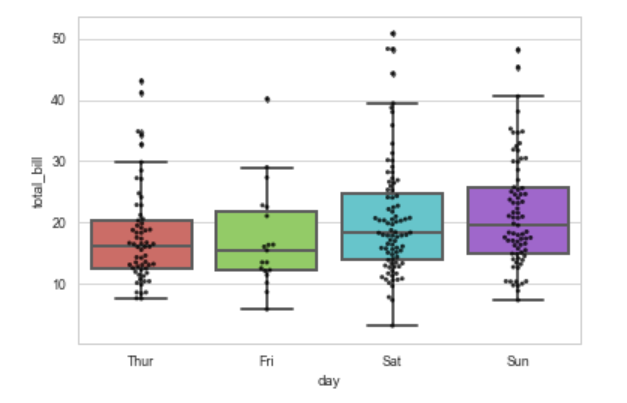通过hue参数再分类

# 绘制箱型图
sns.boxplot(x='day', y='total_bill', data=tips, hue='smoker', palette='Reds')

# 可以添加散点图
sns.swarmplot(x='day', y='total_bill', data=tips, color='k', size=3, alpha=0.8)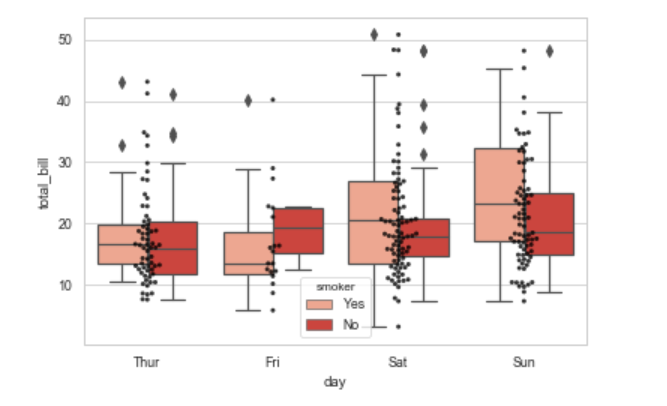### 小提琴图：violinplot()

# 用法和boxplit类似
sns.violinplot(x='day', y='total_bill', data=tips,
linewidth=2,  # 线宽
width=0.8,  # 箱之间的间隔比例
palette='hls',  # 设置调色板
order=['Thur', 'Fri', 'Sat', 'Sun'],  # 筛选类别
scale='area',  # 测度小提琴的宽度：area-面积相同，count-按照样本数量决定宽度，width-宽度一样
gridsize=50,  # 设置小提琴边线的平滑度，越高越平滑，一般可以不设置
inner='box',  # 设置内部显示类型 -> box,  quartile,  point,  stick,  None
# bw=0.8   # 控制拟合程度，一般可以不设置
)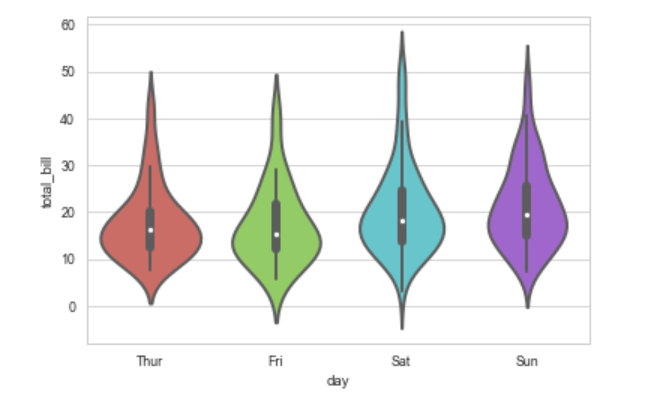通过hue参数再分类

sns.violinplot(x='day', y='total_bill', data=tips, hue='smoker', palette='muted', split=True, # 设置是否拆分小提琴图
inner='quartile')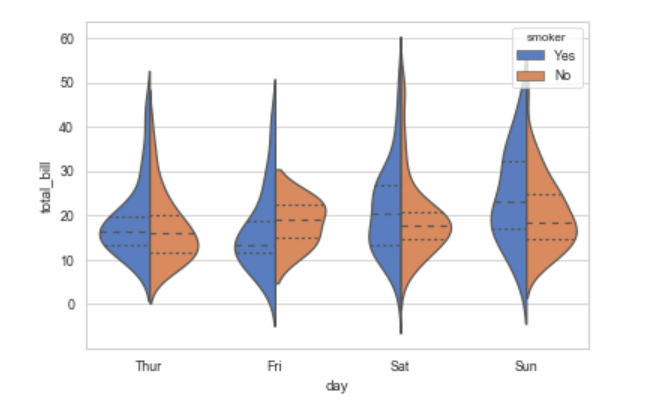结合散点图

sns.violinplot(x='day', y='total_bill', data=tips,  palette='hls',inner=None)
# 插入散点图
sns.swarmplot(x='day', y='total_bill', data=tips,color='w', alpha=0.5 )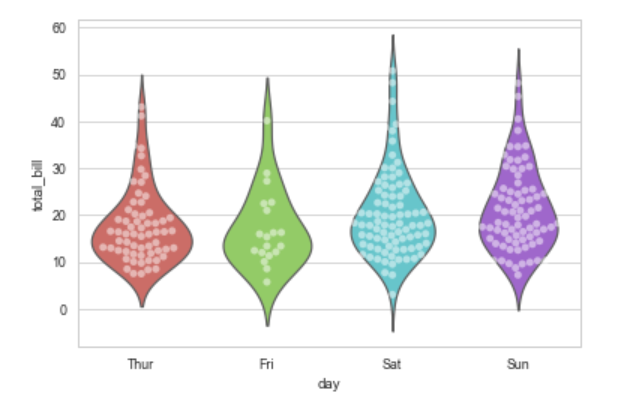### LV图表：lvplot()

# 绘制LV图
sns.lvplot(x='day', y='total_bill', data=tips, palette='mako',
width=0.8,
linewidth=12,
scale='area',  # 设置框的大小 -> linear、exonential、area
k_depth='proportion'  # 设置框的数量 -> proportion、tukey、trustworthy
)

# 可以添加散点图
sns.swarmplot(x='day', y='total_bill', data=tips, color='k', size=3, alpha=0.8)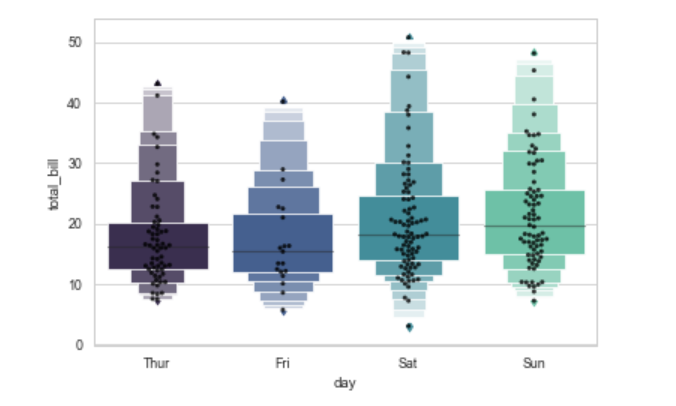展开全文• 今天使用python画了几个好玩的3D展示，现在分享给大家。 先贴上图片 使用的python工具包为： from matplotlib import pyplot as plt import numpy as np from mpl_toolkits.mplot3d import Axes3D 在贴代码...
• 概率分布表示样本数据的模样，长的好不好看如果有图像展示出来就非常完美了，使用Python绘制频率分布直方非常简洁，因为用的频次非常高，这里记录下来。还是Python大法好，代码简洁不拖沓~ 如果数据取值的范围跨度...
• Python绘制正态分布图，求分位数

## 1.绘制正态分布图# ====================python绘制标准正态分布曲线====================
import numpy as np
import math
import matplotlib.pyplot as plt

def gd(x, mu=0, sigma=1):
"""根据公式，由自变量x计算因变量的值

Argument:
x: array
输入数据（自变量）
mu: float
均值
sigma: float
方差
"""
left = 1 / (np.sqrt(2 * math.pi) * np.sqrt(sigma))
right = np.exp(-(x - mu)**2 / (2 * sigma))
return left * right

if __name__ == '__main__':
#  自变量
x = np.arange(-4, 5, 0.1)
#  因变量（不同均值或方差）
y_1 = gd(x, 0, 0.2)
y_2 = gd(x, 0, 1.0)
y_3 = gd(x, 0, 5.0)
y_4 = gd(x, -2, 0.5)

#  绘图
plt.plot(x, y_1, color='green')
plt.plot(x, y_2, color='blue')
plt.plot(x, y_3, color='yellow')
plt.plot(x, y_4, color='red')
#  设置坐标系
plt.xlim(-5.0, 5.0)
plt.ylim(-0.2, 1)

ax = plt.gca()
ax.spines['right'].set_color('none')
ax.spines['top'].set_color('none')
ax.xaxis.set_ticks_position('bottom')
ax.spines['bottom'].set_position(('data', 0))
ax.yaxis.set_ticks_position('left')
ax.spines['left'].set_position(('data', 0))

plt.legend(labels=['$\mu = 0, \sigma^2=0.2$', '$\mu = 0, \sigma^2=1.0$', '$\mu = 0, \sigma^2=5.0$', '$\mu = -2, \sigma^2=0.5$'])
plt.show()


## 2. 基于正态分布求分位数

例子：计算均值为0，标准差为2的正态分布的累积分布为0.1，0.2，0.8，0.9的分位数对应的x分别为多少

from scipy.stats import norm

ppf_list = norm.ppf(q=[0.1, 0.2, 0.8, 0.9], loc=0, scale=2)
print(ppf_list)


输出

[-2.56310313 -1.68324247 1.68324247 2.56310313]

## 3.σ置信区间填充颜色import numpy as np
import matplotlib.pyplot as plt

##制造1000个随机数据
x = np.linspace(60,260,1000)
cigema = 20
miu = 172
#绘制σ=20,μ=172的正态分布
fx = 1 / (cigema * (2 * np.pi)**0.5) * np.exp(-(x - miu)**2 / (2 * cigema**2))
plt.plot(x,fx,color='dodgerblue')
#取μ ~ 1σ的区间
fanwei = x[(x>miu) & (x<miu+cigema)]
#该范围对应的fx
fx2 = 1 / (cigema * (2 * np.pi)**0.5) * np.exp(-(fanwei - miu)**2 / (2 * cigema**2))
#该范围内的曲线与x轴之间的颜色填充
y = np.zeros(fanwei.size)
plt.fill_between(fanwei,fx2,y,fx2 > y,alpha=0.6,color='dodgerblue')
plt.show()


参考链接
 Python绘制正态分布曲线 2022.6
 正态分布置信区间填充颜色 2019.4
 python求正态分布的分位数 2021.7

展开全文分位数 matplotlib
• ## Python绘图总结(seaborn篇)之数据分布

万次阅读 多人点赞 2018-05-15 10:30:48
# 隐藏数据趋势kde，显示数据紧密度fit sns.distplot(x, kde= False , fit=stats.gamma) # 隐藏直方 hist=False sns.distplot(x, hist= False , rug= True ) # rug=True表示显示 rugplot(x) 2、...seaborn
• ##模块导入 import numpy as np import pandas as pd import matplotlib.pyplot as plt ...##数据准备 df = pd.read_csv('/Users/chenxiaogang/Desktop/data.csv',encoding = 'gb2312') data = pd.Series(df['s
• ## Python绘制高斯分布图像

千次阅读 多人点赞 2021-12-03 11:17:19机器学习 开发语言 问题解决
• 折线plt.figure(figsize=(40, 40)) # 确定图像画布的大小plt.subplot(211) # 将画布分为两行一列plt.xlabel('Number of sample', fontsize=40) # x轴的labelplt.ylabel('Char...
• ## 在python中画正态分布图像

万次阅读 多人点赞 2018-01-24 18:06:08
1.正态分布简介 正态分布(normal distribtution)又叫做高斯分布(Gaussian distribution)，是一个非常重要也非常常见的连续概率分布。正态分布大家也都非常熟悉，下面做一些简单的介绍。 假设随机变量X服从一个位置...
• Python绘制概率分布直方 文章目录Python绘制概率分布直方一、代码1、案例一2、案例二二、运行效果1、案例一2、案例二 一、代码 1、案例一 import random import numpy as np import matplotlib.pyplot as plt X...深度学习 机器学习
• 出手写数字图片的数据分布图 from time import time import numpy as np import matplotlib . pyplot as plt from sklearn import datasets from sklearn . manifold import TSNE import ...数据处理
• Xmax) plt.ylabel(Ylabel) plt.ylim(Ymin,Ymax) plt.title(Title) plt.show() draw_hist(areaList,'AreasList','Area','number',50.0,250,0.0,8) # 直方展示 draw_hist(perimeterList,'perimeterList','Area','...
• 今天小编就为大家分享一篇Python数据可视化:幂律分布实例详解，具有很好的参考价值，希望对大家有所帮助。一起跟随小编过来看看吧
• Python绘制高斯分布（正态分布）图像，附python绘图技巧
• 正态分布和置信区间 正态分布（Normal Distribution）又叫高斯分布，是一种非常重要的概率分布。其概率密度函数的数学表达如下： 置信区间是对该区间能包含未知参数的可置信的程度的描述。 使用SciPy求解置信区间 ...
• 一维高斯分布图像 import math import numpy as np from matplotlib import pyplot as plt def gaussian_distibution(center=50, sigma=1, length = 100): data = np.array(list(range(length))) data = np.exp...
• 先来看下数据的类型，常见的数据分类方式有三种：第一种是按照数据的结构属性分类，根据数据的存储形式分为结构化数据和非结构化数据，例如数据库的存储对象基本上都是结构化数据，结构化数据是进行数据分析的基本...
• 我们平时做数据分析的时候，经常要了解数据分布情况，这时候就需要出频率分布直方，博主采用的画图工具是python中的seaborn，它的画图效果比matplotlib要好 。 首先需要明确一下直方和条形的区别：...
• 不过，恋习python突然想到，可以通过python将故宫的建筑物图片，转化为手绘（素描效果）。 效果如下：? 一、概念与原理我们都知道手绘效果的特征...根据灰度变化来模拟人类视觉的模拟程度把图像看成二维离散...
• 用散点可以直观的查看数据分布情况。matplotlib模块的pyplot有散点的函数，但是该函数要求x轴是数字类型。pandas的plot函数里，散点类型'scatter'也要求数字型的，用时间类型的会报错。在搜索阅读了几十篇...
• 这篇经验告诉你，如何利用python的matplotlib模块直方。工具/原料windows系统电脑一台python软件，并安装matplotlib模块spyder编辑器方法/步骤1第一步，点击键盘 win+r，打开运行窗口；在窗口中输入“cmd"，点击...
• 分布是用来描述事件...#寻找真知派#图1 考试成绩分布图(正态分布)事件的分布类型有很多种，比如指数分布、t分布、泊松分布等，每种分布都对应于一个概率密度函数(连续随机变量)或概率质量函数(离散随机变量)。通......